# Bipolar Stepper Motors (Part II): Microstepping and Decay ModesGet valuable resources straight to your inbox - sent out once per month

## Introduction

In Part I of this series, we covered the bipolar stepper motor’s three control modes as well as its mechanical structure, including dual full-bridge drive. In Part II, we will discuss microstepping and how it controls the dual full-bridge drive.

## Microstepping

Previously, half-step stepping mode was introduced as a combination of single-phase stepping and full-step stepping to obtain more electrical angle positions. Microstepping adds intermediate angle positions, which creates finer steps.

Figure 1 shows eight subdivisions in microstepping. The 90° electrical angle for single-phase stepping is divided into eight equal parts, representing eight current positions. The current at each position is a vector synthesized by the current of the phase A winding and phase B winding. The amplitude of the resulting vectors is always 1.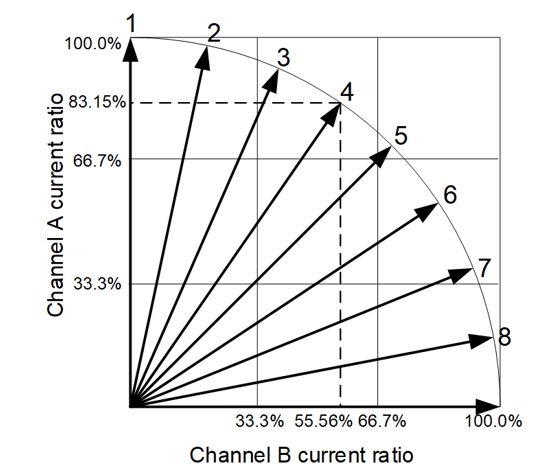Figure 1: Eight Segments of Electrical Angle

The current at each position must be projected onto phase A and phase B, respectively, to obtain the control value (see Table 1).

Table 1: Values for Eight-Step Microstepping

 Stepping Mode Relative Current Level Sequence (%) Current Position Phase A Phase B Eighth-step 100 0 1 98.08 19.51 2 92.39 38.27 3 83.15 55.56 4 70.71 70.71 5 55.56 83.15 6 38.27 92.39 7 19.51 98.08 8

#### Related Content

The phase A and phase B currents can be controlled based on the corresponding values in Table 1. By controlling the current, it is possible to synthesize the current vectors for phase A and phase B at the corresponding angle.

Under the control of eight subdivisions (or steps), the current of the bipolar stepper motor resembles a sine wave (see Figure 2). If there are more steps, the current through the bipolar stepper motor windings resembles a standard sinusoidal wave. The sinusoidal current waveform reduces motor output torque fluctuations and forms a circular rotating magnetic field in space, which improves the stepping motor’s rotational stability.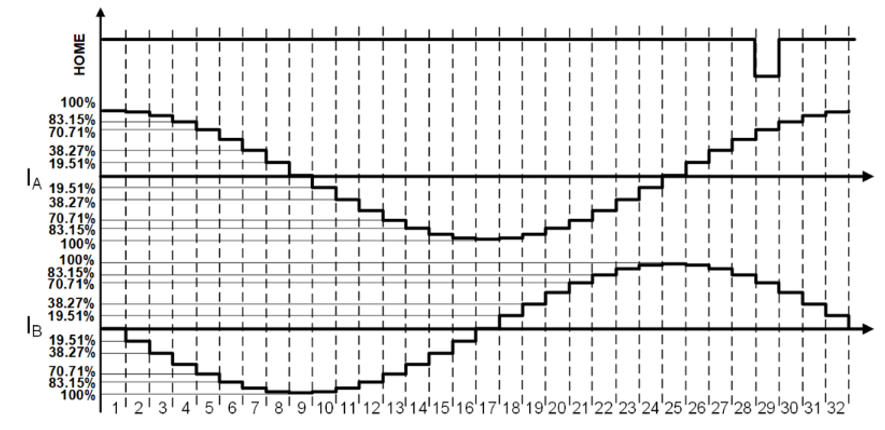Figure 2: Phase A and Phase B Current Waveform under Eight-Step Microstepping

## Current Regulation with Decay Modes

Slow decay and fast decay are the typical control methods used to stabilize the current at each step. Consider a singular step on phase A. Figure 3 shows phase A’s current regulation waveform, which is achieved by controlling the on/off function of the four MOSFETs driven by phase A’s full-bridge drive.

The two decay modes will be discussed in further detail below.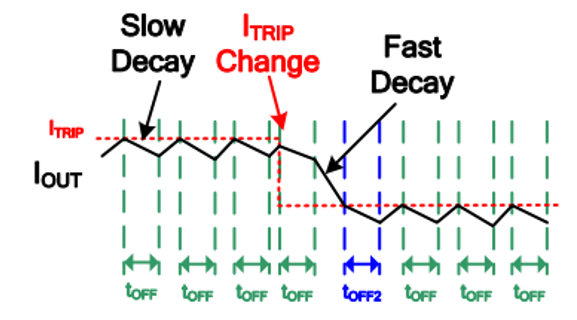Figure 3: Phase A's Current Regulation Waveform

## Slow Decay

When Q1 and Q4 turn on, the supply voltage (U) is applied to phase A’s winding, and the current starts to rise. In the corresponding equivalent circuit, R is the winding resistance and L is the inductance (which have equivalent impedances), and E is the back EMF generated in the winding by the rotor’s moving magnetic field (see Figure 4). The reverse electromotive force acts on the winding circuit.

Once the current value reaches the desired value, the current must be reduced. Otherwise, the current may continue to increase and exceed its set value. In this scenario, slow decay is required.

Figure 4 shows the slow decay process for phase A’s full-bridge drive.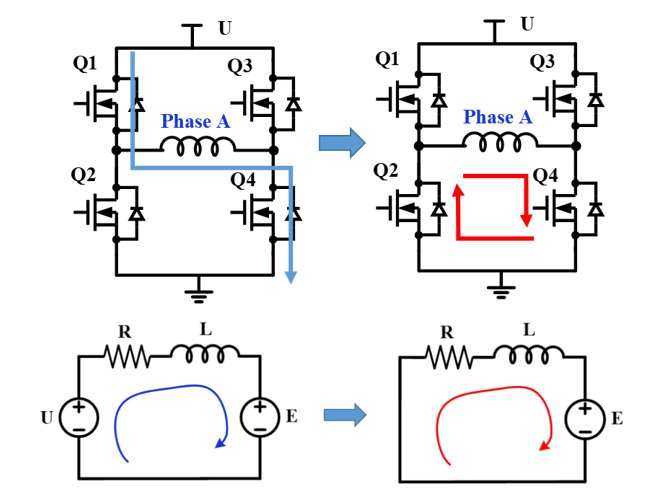Figure 4: Slow Decay Process for Phase A's Full-Bridge Drive

To enter slow decay, turn off Q1 and turn on Q2 (ignoring the dead time). This is equivalent to short-circuiting the phase A winding. Due to the current through the winding inductance, the current direction does not abruptly change, and the current forms a circulating current in the two lower MOSFETs. Only the back electromotive force (E) acts on the circuit, and the current is subject to a reverse voltage drop (-E) and starts to drop. If the voltage drop caused by the resistance is disregarded, the current decreases at the speed of (E / L).

After the current drops for a period of time, Q2 is turned off Q1 is turned on to allow the current to rise again. This process stabilizes the current at each step.

## Fast Decay

When the current is about to enter a step that requires lower current, the current must drop further. If the current does not decrease fast enough, the slow decay speed may not be fast enough to reduce the current to the desired level. In this situation, fast decay is required (See Figure 5).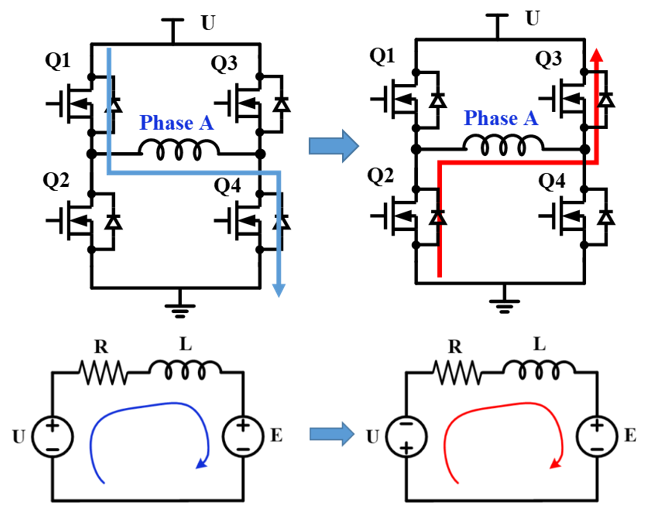Figure 5: Fast Decay Process for Phase A’s Full-Bridge Drive

During fast decay, Q2 and Q3 turn on after Q1 and Q4 turn off (ignoring the dead time). At this time, the supply voltage is applied in reverse to the phase A winding and in series with the back EMF (E). The current flows from Q2 to Q3 because the current through the winding inductance cannot change suddenly. The current decreases at the speed of ((E + U) / L), so the current drops faster than slow decay.

## Comparison of the Different Current Controls

When the current drops to the set value of the following step, the current regulation scheme can change from fast decay to slow decay. Utilizing both slow decay and fast decay allows the controlled current to change quickly while minimizing the current ripple. Figure 6 shows current waveforms that use fast decay, slow decay, and a combination of fast and slow decay, where the current waveform is green.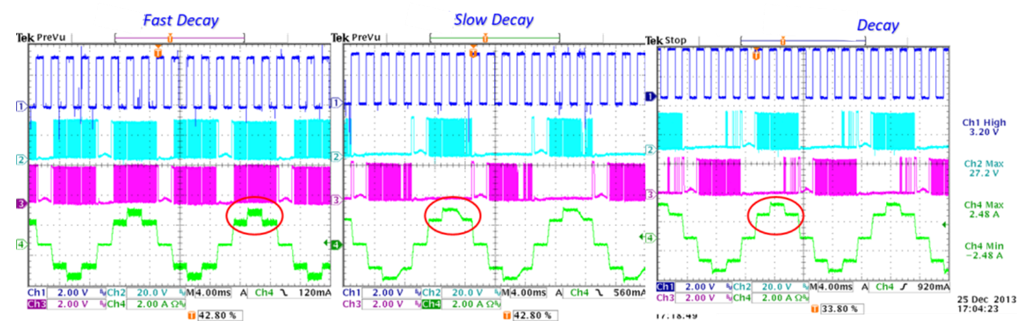Figure 6: Current Waveforms under Different Current Controls

During slow decay, the current decreases over a longer period of time while dropping to the next step, but this results in a distorted waveform.

When fast decay and slow decay work together, slow decay regulates the current when the bipolar stepper motor is held at a step position, while fast decay controls the current more effectively when the current must rapidly drop to the next step value.

## Conclusion

Microstepping allows for finer positioning and smoother operation compared to full-step or half-step mode. This article discussed how slow decay and fast decay between steps can be used to stabilize the current. In addition, combining these decay modes can decrease torque ripple, which reduces vibration and noise.

MPS offers a wide array of stepper motor drivers to meet your application needs.